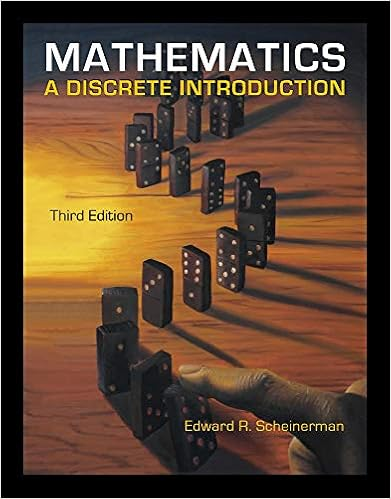# By another edge the edge in the path therefore e

• Test Prep
• 13
• 100% (7) 7 out of 7 people found this document helpful

This preview shows page 8 - 13 out of 13 pages.

##### We have textbook solutions for you!
The document you are viewing contains questions related to this textbook.The document you are viewing contains questions related to this textbook.
Chapter 9 / Exercise 21
Mathematics: A Discrete Introduction
ScheinermanExpert Verified
8
##### We have textbook solutions for you!
The document you are viewing contains questions related to this textbook.The document you are viewing contains questions related to this textbook.
Chapter 9 / Exercise 21
Mathematics: A Discrete Introduction
ScheinermanExpert Verified
15.[10 points]Recall theNP-complete problem SAT: The input is a string representing apropositional formula, such as(¬((x1∨ ¬x2)(x5x10))((x7x8)(¬x9x11))).The answer is YES if there is a truth assignment to the variables appearing in the formula whichmakes the formula true, and NO otherwise.Consider now the MSAT problem. It is the same as the SAT problem, except that the answeris YES if there areat least twotruth assignments which make the formula true, and NO if thereis at most one satisfying truth assignment. Prove that MSAT isNP-complete. YourNP-hardnessreduction should be from SAT.To show that MSAT is in NP, let the proof be two truth assignments, which hasO(n) size. Theverification algorithm first checks that the two assignments are distinct, and then verifies that theyboth satisfy the formula. Clearly, the verification algorithm takesO(n) time.To show that MSAT is NP-hard, we reduce from SAT. LetFbe an input to SAT and consider thefollowing steps :1. Find a variableynot inF2. Create a new formulaF=F(y∨ ¬y)3. Run the MSAT oracle on the new formula, return the answer
9
BONUS [3 points]Fix a positive integerr.Suppose the edges of the complete undirectedgraph onnvertices (i.e.Kn) are colored usingccolors.Show that ifn > rc, there must be amonochromatic path of lengthr.(A monochromatic path is a path in which all the edges arecolored with the same color.)10
•••